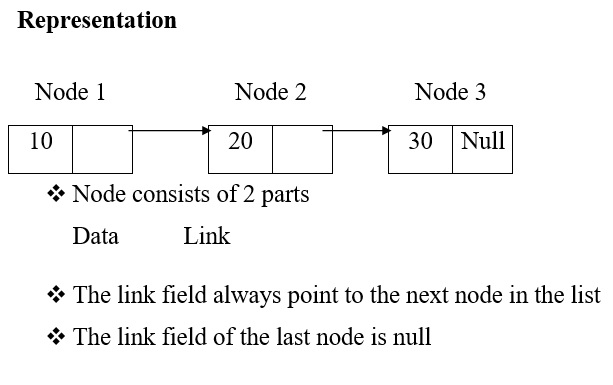# C program to display numbers in reverse order using single linked list

Linked lists use dynamic memory allocation and are collection of nodes.

Nodes have two parts which are data and link.

The types of linked lists in C programming language are as follows −

• Single / Singly linked lists
• Double / Doubly linked lists

The diagram given below depicts the representation of single linked list.## Example

Following is the C program to display the numbers in reverse order by using the single linked list

Live Demo

#include <stdio.h>
#include <stdlib.h>
struct node {
int num;
struct node *nextptr;
}*stnode;
void createNodeList(int n);
void reverseDispList();
void displayList();
int main(){
int n;
printf(" single Linked List : print it in reverse order :");
printf("------------------------------------------------------------------------------");
printf(" Input the number of nodes : ");
scanf("%d", &n);
createNodeList(n);
printf(" Data entered in the list are : ");
displayList();
reverseDispList();
printf(" The list in reverse are : ");
displayList();
return 0;
}
void createNodeList(int n){
struct node *fnNode, *tmp;
int num, i;
stnode = (struct node *)malloc(sizeof(struct node));
if(stnode == NULL) {
printf(" Memory can not be allocated.");
}
else{
// reads data for the node through keyboard
printf(" Input data for node 1 : ");
scanf("%d", &num);
stnode-> num = num;
stnode-> nextptr = NULL;
tmp = stnode;
for(i=2; i<=n; i++){
fnNode = (struct node *)malloc(sizeof(struct node));
if(fnNode == NULL) {
printf(" Memory can not be allocated.");
break;
}
else{
printf(" Input data for node %d : ", i);
scanf(" %d", &num);
fnNode->num = num;
fnNode->nextptr = NULL;
tmp->nextptr = fnNode;
tmp = tmp->nextptr;
}
}
}
}
void reverseDispList(){
struct node *prevNode, *curNode;
if(stnode != NULL){
prevNode = stnode;
curNode = stnode->nextptr;
stnode = stnode->nextptr;
prevNode->nextptr = NULL; //convert the first node as last
while(stnode != NULL){
stnode = stnode->nextptr;
curNode->nextptr = prevNode;
prevNode = curNode;
curNode = stnode;
}
stnode = prevNode; //convert the last node as head
}
}
void displayList(){
struct node *tmp;
if(stnode == NULL){
printf(" No data found in the list.");
}
else{
tmp = stnode;
while(tmp != NULL){
printf(" Data = %d", tmp->num);
tmp = tmp->nextptr;
}
}
}

## Output

When the above program is executed, it produces the following result −

Single Linked List : print it in reverse order :
------------------------------------------------------------------------------
Input the number of nodes : 5
Input data for node 1 : 12
Input data for node 2 : 45
Input data for node 3 : 11
Input data for node 4 : 9
Input data for node 5 : 10

Data entered in the list are :
Data = 12
Data = 45
Data = 11
Data = 9
Data = 10

The list in reverse are :
Data = 10
Data = 9
Data = 11
Data = 45
Data = 12

Updated on: 03-Sep-2021

525 Views